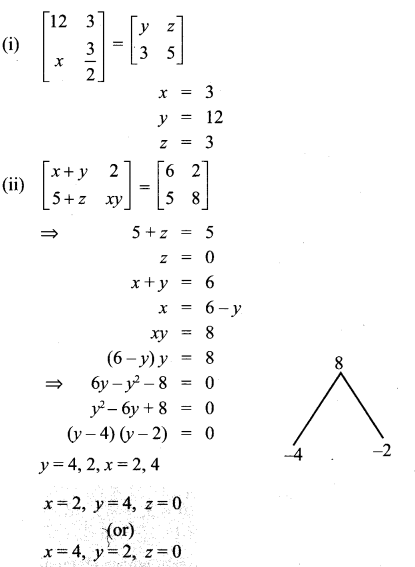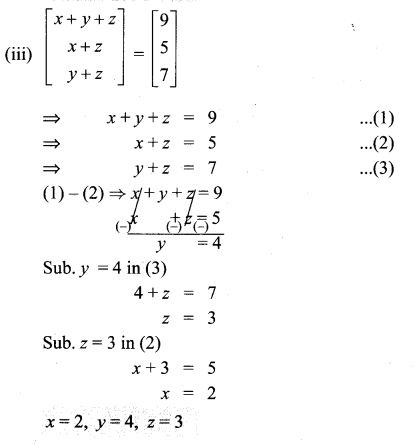## Tamilnadu Samacheer Kalvi 10th Maths Solutions Chapter 3 Algebra Ex 3.16

10th Maths Exercise 3.16 Solution Question 1.
In the matrix A = $$\left[\begin{array}{cccc}{8} & {9} & {4} & {3} \\ {-1} & {\sqrt{7}} & {\frac{\sqrt{3}}{2}} & {5} \\ {1} & {4} & {3} & {0} \\ {6} & {8} & {-11} & {1}\end{array}\right]$$
(i) The number of elements
(ii) The order of the matrix
(iii) Write the elements a22, a23, a24, a34, a43, a44
Solution:
(i) 16
(ii) 4 × 4
(iii) $$\sqrt{7}, \frac{\sqrt{3}}{2}$$, 5, 0, -11, 1

10th Maths Exercise 3.16 Solutions Question 2.
If a matrix has 18 elements, what are the possible orders it can have? What if it has 6 elements?
Solution:
1 × 18, 2 × 9, 3 × 6, 6 × 3, 9 × 2, 18 × 1 and 1 × 6, 2 × 3, 3 × 2, 6 × 1

10th Maths Exercise 3.16 Answers Question 3.
Construct a 3 × 3 matrix whose elements are given by then find the transpose of A.
(i) aij = |i – 2j|
(ii) aij = $$\frac{(i+j)^{3}}{3}$$
Solution:
(i) aij = |i – 2j|
a11 = |1 – 2 × 1| = |1 – 2| = |-1| = 1
a12 = |1 – 2 × 2| = |1 – 4| = |-3| = 3
a13 = |1 – 2 × 3| = |1 – 6| = |-5| = 5
a21 = |2 – 2 × 1| = |2 – 2| = 0
a22 = |2 – 2 × 2| = |2 – 4| = |-2| = 2
a23 = |2 – 2 × 3| = |2 – 6| = |-4| = 4
a31 = |3 – 2 × 1| = |3 – 2| = |1| = 1
a32 = |3 – 2 × 2| = |3 – 4| = |-1| = 1
a33 = |3 – 2 × 3| = |3 – 6| = |-3| = 3
∴ $$\left[\begin{array}{lll}{1} & {3} & {5} \\ {0} & {2} & {4} \\ {1} & {1} & {3}\end{array}\right]$$ is the required 3 × 3 matrix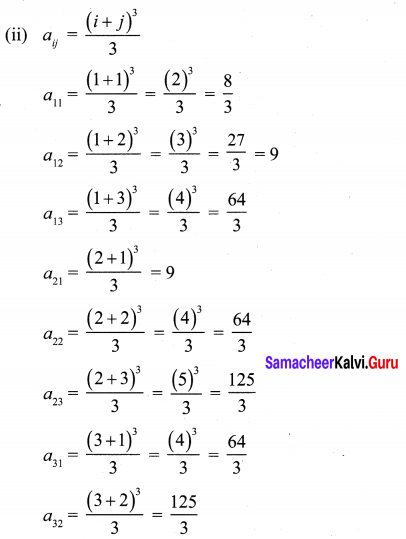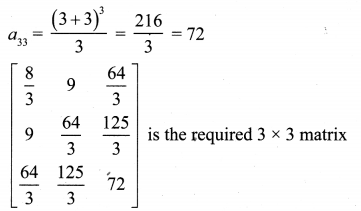Ex 3.16 Class 10 Samacheer Question 4.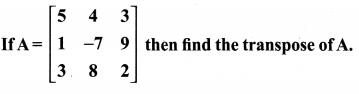Solution: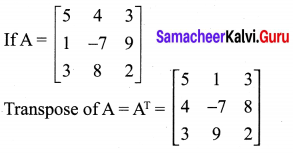10th Maths Exercise 3.16 Question 5.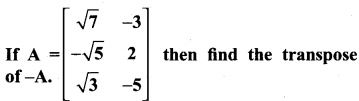Solution: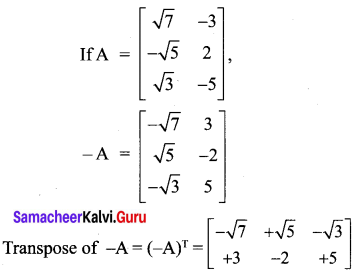10th Maths 3.16 Question 6.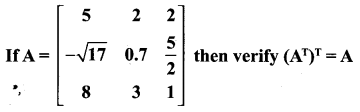Solution: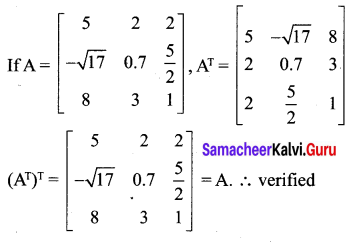Exercise 3.16 Class 10 Question 7.
Find the values of x,y and z from the following equations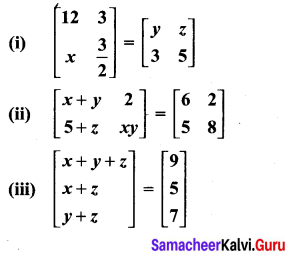Solution: